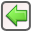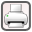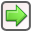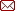﻿ Module 1 - Energy Accounting > Accounts & Invoices Tab > User Defined Components > Functions > SumOfTiers
 SumOfTiersrev. 2011-02-09SumOfTiers

Description:

Calculates the total cost for a tiered rate structure.

Syntax:

SumOfTiers(quantity, tier1UnitCost, tier1UpperLimit, tier2UnitCost, tier2UpperLimit,..., tierXUnitCost, [tierXUpperLimit])

Type:

Double Precision Numeric

Arguments:

 Argument Type Description quantity Numeric The quantity (ie Consumption/Demand etc) tier1UnitCost Numeric The unit cost within tier 1 tier1UpperLimit Numeric The upper quantity limit for tier 1. tier2UnitCost Numeric The unit cost within tier 2 tier2UpperLimit Numeric The upper quantity limit for tier 2. tierXUnitCost Numeric The unit cost within tier X [tierXUpperLimit] Numeric The upper quantity limit for tier X This is an optional field. If it is not specified it is assumed there is no upper limit.

Examples:

Consider an electricity tariff which includes three distinct rate tiers for the various levels of consumption.

 • 0 to 2,500 kWh: \$0.09/kWh
 • 2,500 to 10,000 kWh: \$0.07/kWh
 • 10,000 kWh onwards:  \$0.05/kWh

Assuming 7500 kWh were consumed the total cost can be calculated using the SumOfTiers function.

 Expression Result SumOfTiers(7500, 0.09, 2500, 0.07, 10000, 0.05,100000) 575